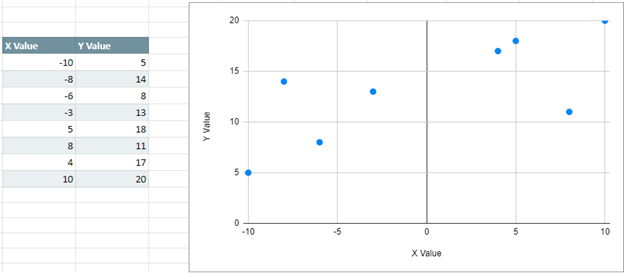# Move Vertical Axis to the Left – Excel & Google Sheets

This tutorial will demonstrate how to move the vertical axis to the left of the graph in Excel and Google Sheets.

## Move Vertical Axis (Y Axis) to Left of Graph in Excel

When working with data where the X Axis has negative values in it, you’ll see that the Y Axis automatically appears in the middle of the graph. This tutorial will show how to move it to the left of the graph so that it can be seen easier to see.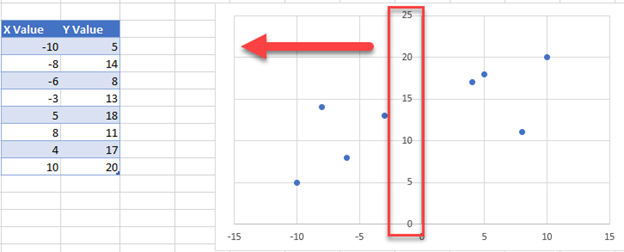### Moving Y Axis to the Left of the Graph

1. Click on the Y Axis
2. Select Format Axis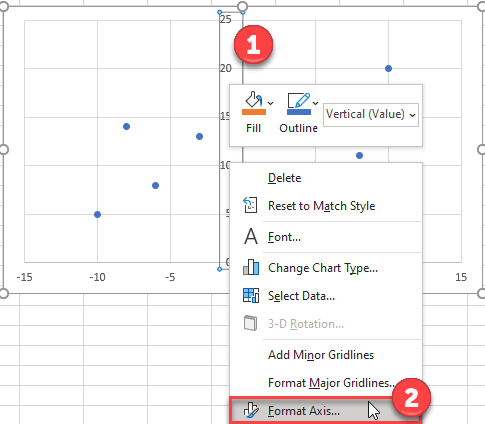3. Under Format Axis, Select Labels

4. In the box next to Label Position, switch it to Low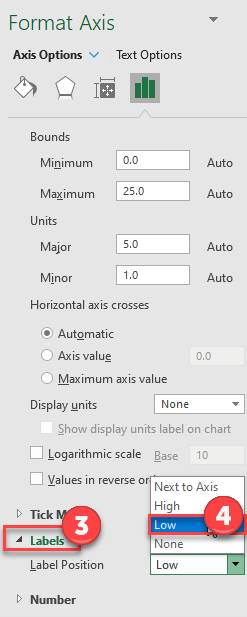### Final Graph

Now your Y Axis Labels are showing at the left of the graph instead of in the middle, which is clearer to see the labels.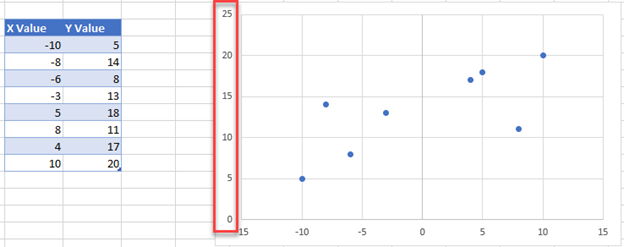## Move Vertical Axis to Left in Google Sheets

Unlike Excel, Google Sheets will automatically put the Y Axis values at the left of the sheet. Your graph should automatically look like the one below.